# Consider the matrix A and the reduced row echelon form of A. A 1 -2 -2...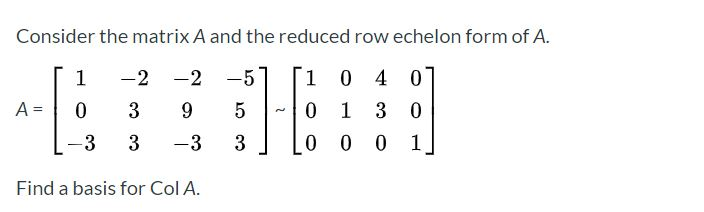Consider the matrix A and the reduced row echelon form of A. A 1 -2 -2 0 39 -3 3 -3 1 0 4 0 0 1 3 0 0 0 0 1 Find a basis for Col A.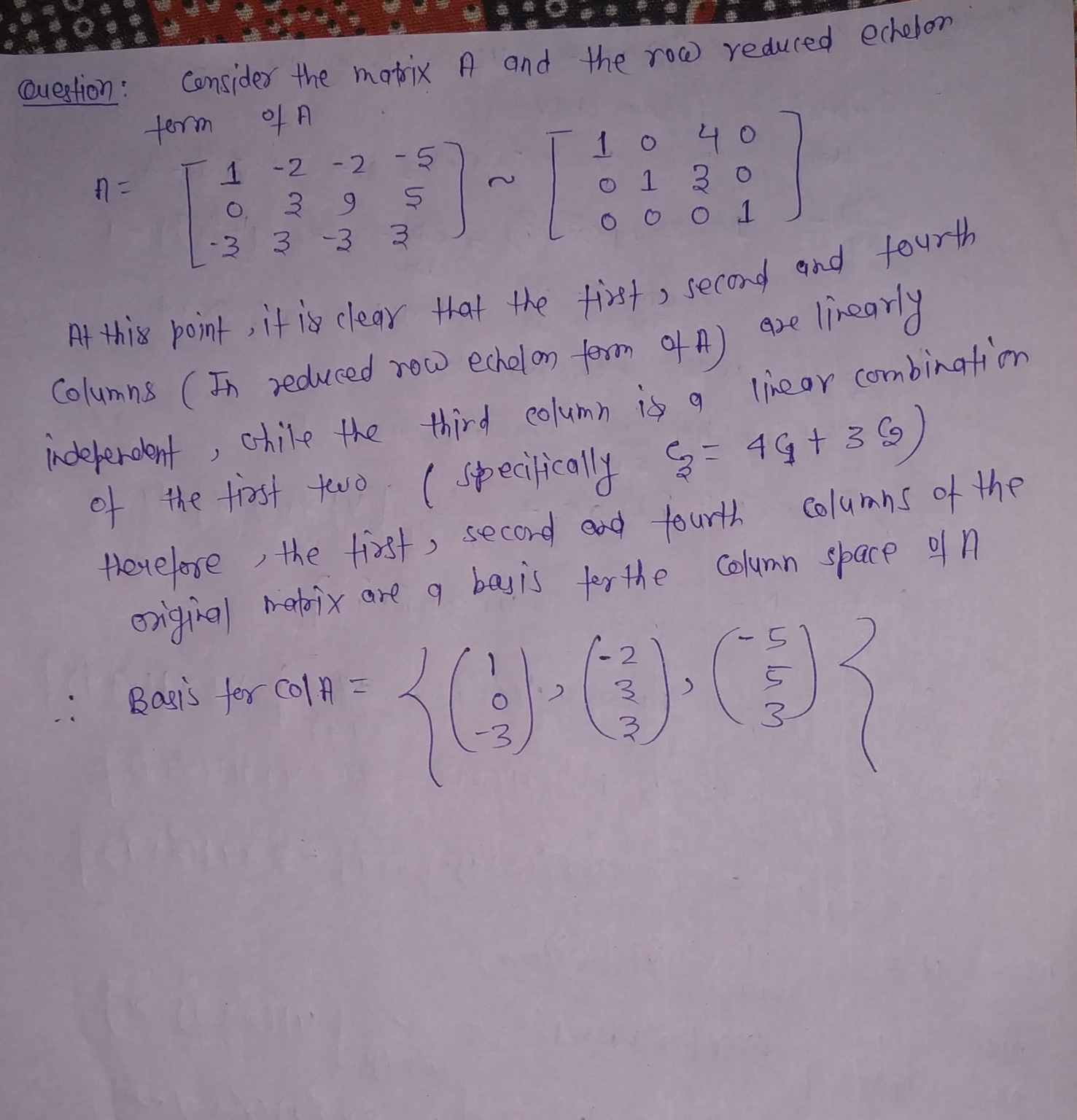##### Add Answer of: Consider the matrix A and the reduced row echelon form of A. A 1 -2 -2...
Similar Homework Help Questions
• ### Consider the matrix A and the reduced row echelon form of A. 1 -2 -5 [1...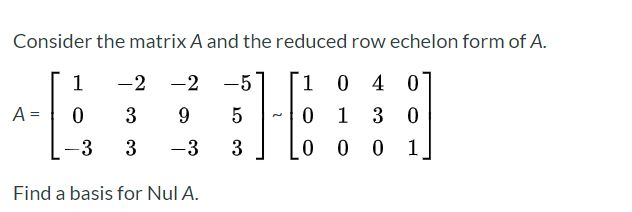Consider the matrix A and the reduced row echelon form of A. 1 -2 -5 [1 -2 9 0 4 0 1 3 0 A= 0 3 5 0 -3 3 -3 3 0 0 0 1 Find a basis for Nul A.

• ### 4. Give the row-echelon form and the reduced row-echelon form of the matrix: A = 11...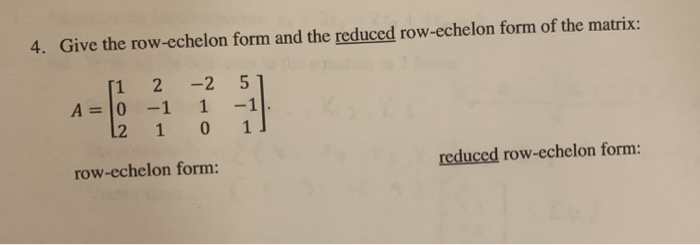4. Give the row-echelon form and the reduced row-echelon form of the matrix: A = 11 2 0 -1 12 1 -2 51 1 -1 0 1] row-echelon form: reduced row-echelon form:

• ### 1 1. The matrix A and it reduced echelon form B are given below. 1 -2...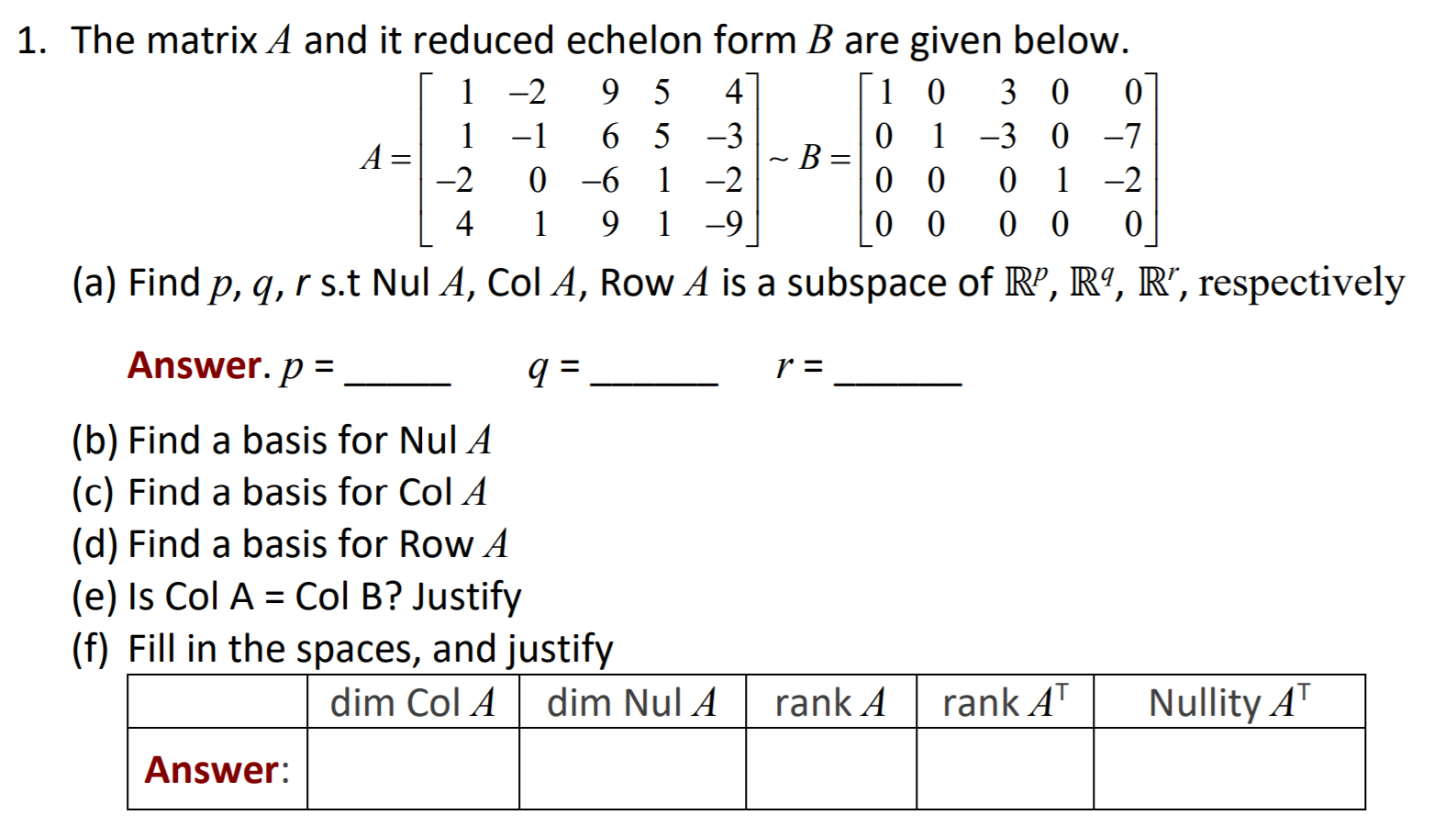1 1. The matrix A and it reduced echelon form B are given below. 1 -2 9 5 4 1 0 3 0 0 -1 6 5 -3 0 1 -3 0 -7 A= ~B= -2 0 -6 1 -2 0 0 1 -2 4 9 1 -9 0 0 0 0 0 (a) Find p, q, r s.t Nul A, Col A, Row A is a subspace of RP, R9, R”, respectively o 1 Answer. p = a =...

• ### 4 1 1. The matrix A and it reduced echelon form B are given below. 1-2...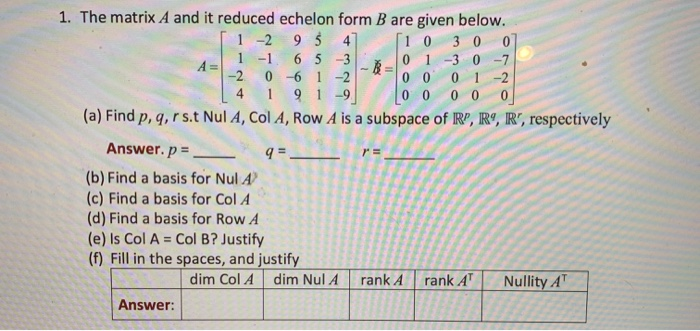4 1 1. The matrix A and it reduced echelon form B are given below. 1-2 95 4 10 3 0 0 1 -1 6 5 3 0 1 -3 0 -7 -2 0 -6 1 -2 0 0 0 1 -2 9 1-9 0 0 0 0 0 (a) Find p, q, r s t Nul A, Col A, Row A is a subspace of RP, R9, R', respectively Answer. p = 9= (b) Find a basis for Nul...

• ### 4 1 1. The matrix A and it reduced echelon form B are given below. 1-2...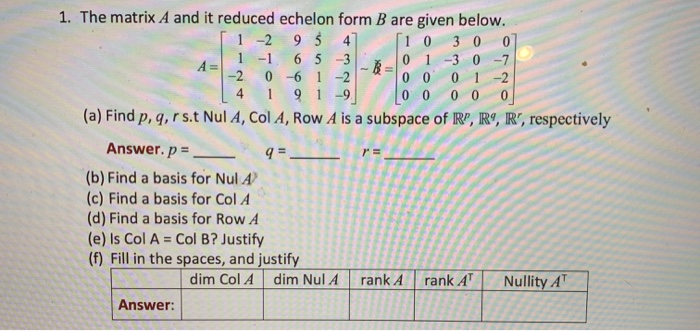4 1 1. The matrix A and it reduced echelon form B are given below. 1-2 95 4 10 3 0 0 1 -1 6 5 3 0 1 -3 0 -7 -2 0 -6 1 -2 0 0 0 1 -2 91-9 0 0 0 0 0 (a) Find p, q, rs. Nul A, Col A, Row A is a subspace of R", R9, R', respectively Answer. p = 9=- (b) Find a basis for Nul A (c) Find...

• ### 1. The matrix A and it reduced echelon form B are given below. 1-2 95 4...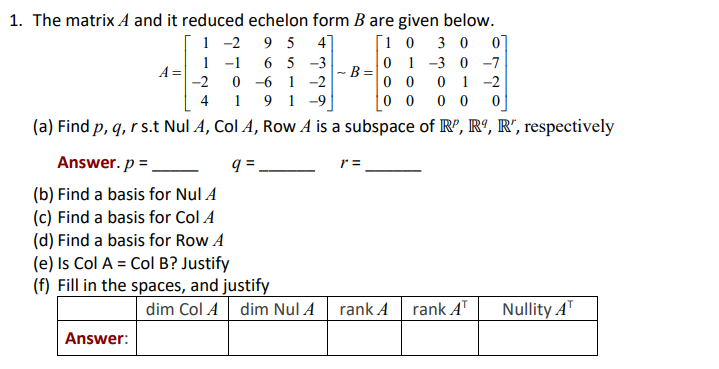1. The matrix A and it reduced echelon form B are given below. 1-2 95 4 [1 0 3 0 0 1 -1 6 5 -3 A= 0 1 -3 0 -7 -B= -2 0 -6 1 -2 0 0 0 1 -2 4 9 -9 0 0 0 0 0 (a) Find p, q, rs. Nul A, Col A, Row A is a subspace of R”, R9, R", respectively Answer.p = 9. r = (b) Find a basis for...

• ### 4. Consider the matrix 0- 3 1 -2 1 4 (a) Use Matlab to determine the reduced row echelon form of A (b) If v, V2, vs, v4...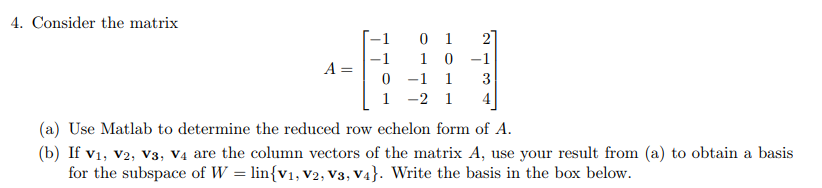4. Consider the matrix 0- 3 1 -2 1 4 (a) Use Matlab to determine the reduced row echelon form of A (b) If v, V2, vs, v4 are the column vectors of the matrix A, use your result from (a) to obtain a basis for the subspace of W-linsv1, V2, V3, V4. Write the basis in the box below 4. Consider the matrix 0- 3 1 -2 1 4 (a) Use Matlab to determine the reduced row echelon form...

• ### Use row-reduction to put the following matrix to reduced row echelon form. 1 5 4 2...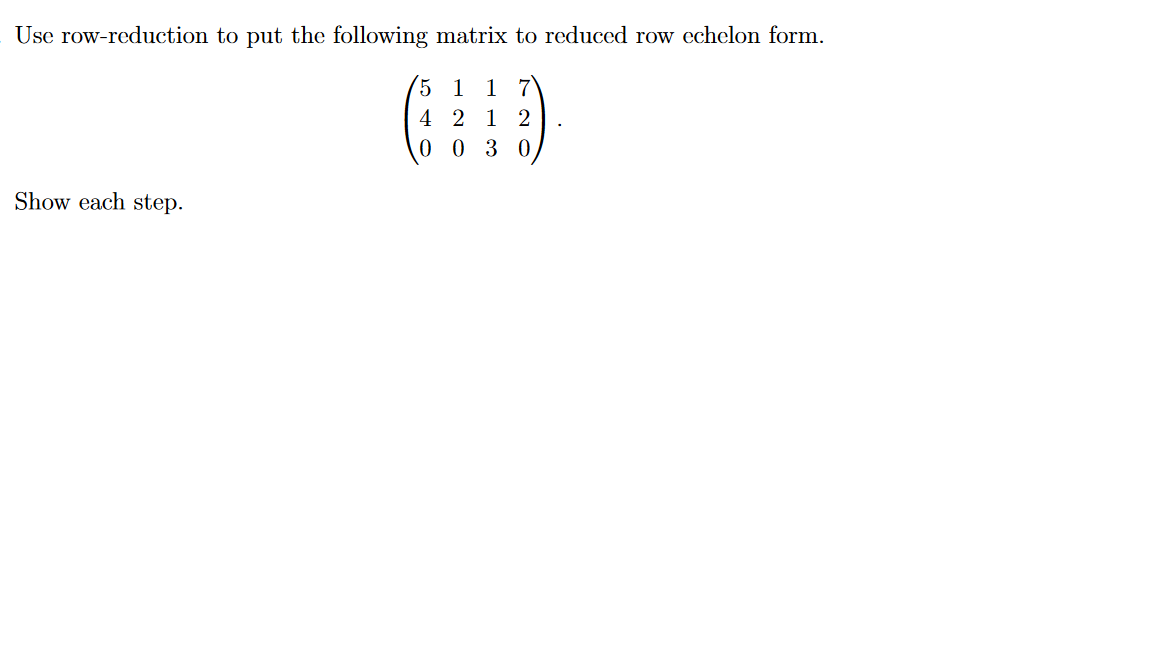Use row-reduction to put the following matrix to reduced row echelon form. 1 5 4 2 1 2 0 0 3 0 Show each step.

• ### Question 3 Use row-reduction to put the following matrix to reduced row echelon form. 5 1...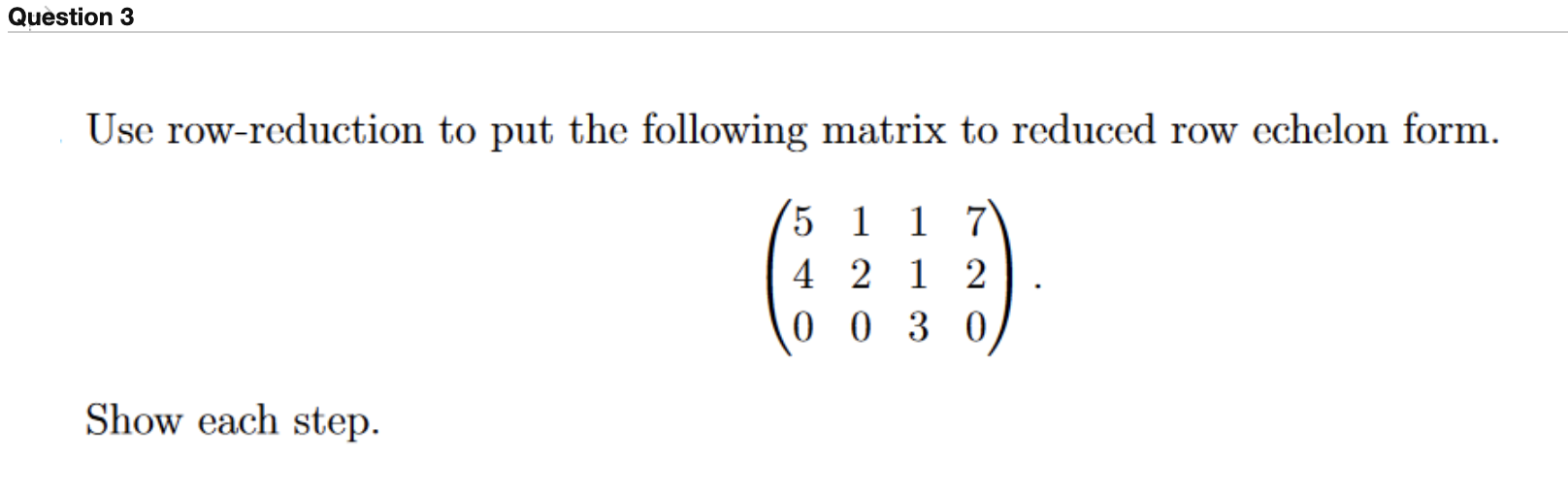Question 3 Use row-reduction to put the following matrix to reduced row echelon form. 5 1 1 7 4 2 1 2 0 0 3 0 Show each step.

• ### Section 1.2 Row Echelon Form: Problem 6 Previous Problem Problem ListNext Problem (1 point) Solve the system by finding the reduced row-echelon form of the augmented matrix. reduced row-echelon form...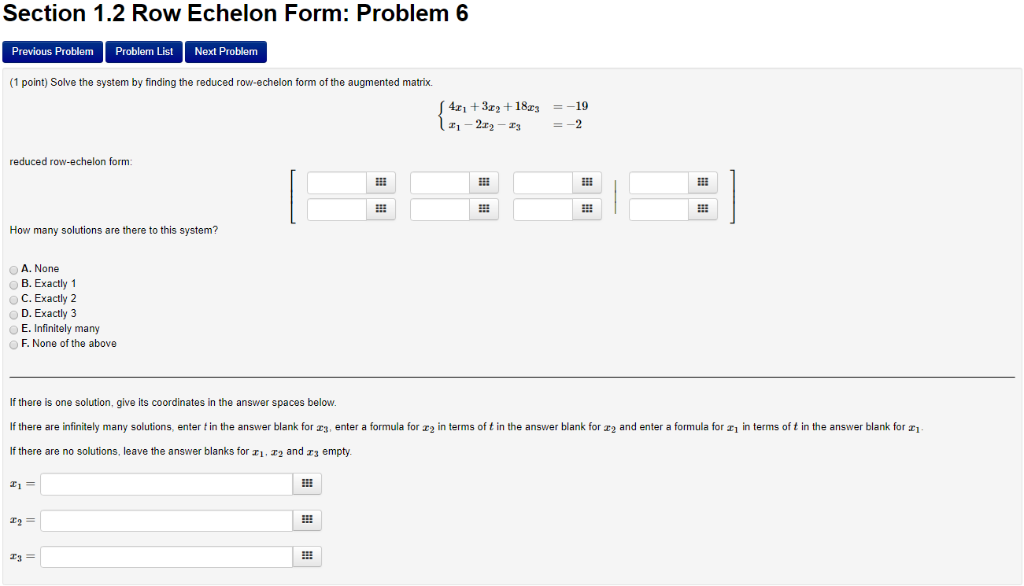Section 1.2 Row Echelon Form: Problem 6 Previous Problem Problem ListNext Problem (1 point) Solve the system by finding the reduced row-echelon form of the augmented matrix. reduced row-echelon form How many solutions are there to this system? A. None B. Exactly C.Exactly 2 D. Exactly 3 E. Infinitely many F. None of the above If there is one solution, give its coordinates in the answer spaces below. If there are infinitely many solutions, enter in the answer blank for...

Free Homework App